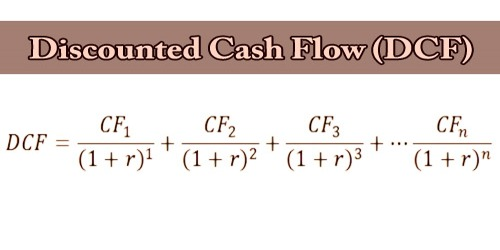Finance

# Discounted Cash Flow (DCF)In finance, discounted cash flow (DCF) is a valuation method used to estimate the value of an investment based on its future cash flows. DCF analysis attempts to figure out the value of an investment today, based on projections of how much money it will generate in the future. This applies to both financial investments for investors and for business owners looking to make changes to their businesses, such as purchasing new equipment.

Discounted cash flow (DCF) analysis is widely used in investment finance, real estate development, corporate financial management, and patent valuation. It was used in industry as early as the 1700s or 1800s, widely discussed in financial economics in the 1960s, and became widely used in U.S. courts in the 1980s and 1990s.

To apply the method, all future cash flows are estimated and discounted by using the cost of capital to give their present values (PVs). The sum of all future cash flows, both incoming and outgoing, is the net present value (NPV), which is taken as the value of the cash flows in question. For further context see Valuation overview; and for the mechanics see Valuation using discounted cash flows, which includes modifications typical for startups, private equity and venture capital, corporate finance “projects”, and mergers and acquisitions.

The purpose of DCF analysis is to estimate the money an investor would receive from an investment, adjusted for the time value of money. The time value of money assumes that a dollar today is worth more than a dollar tomorrow because it can be invested. As such, a DCF analysis is appropriate in any situation where a person is paying money in the present with expectations of receiving more money in the future.

For example, assuming a 5% annual interest rate, \$1.00 in a savings account will be worth \$1.05 in a year. Similarly, if a \$1 payment is delayed for a year, its present value is \$.95 because it cannot be put in our savings account to earn interest.

Using DCF analysis to compute the NPV takes as input cash flows and a discount rate and gives as output a present value. The opposite process takes cash flows and a price (present value) as inputs, and provides as output the discount rate; this is used in bond markets to obtain the yield.

Discounted Cash Flow (DCF) Formula –

The discounted cash flow (DCF) formula is equal to the sum of the cash flow in each period divided by one plus the discount rate (WACC) raised to the power of the period number.

Here is the DCF formula:

DCF = CF1/(1+r)1 + CF2/(1+r)2 + CF3/(1+r)3 + … + CFn/(1+r)n

Where:

CF = the cash flow for the given year.

r = the interest rate or discount rate

n = the period number

Analyzing the Components of the Formula:

Cash Flow (CF) – Cash Flow (CF) represents the free cash payments an investor receives in a given period for owning given security (bonds, shares, etc.)

When building a financial model of a company, the CF is typically what’s known as unlevered free cash flow.  When valuing a bond, the CF would be interest and or principal payments.

Discount Rate (r) – For business valuation purposes, the discount rate is typically a firm’s Weighted Average Cost of Capital (WACC).  Investors use WACC because it represents the required rate of return that investors expect from investing in the company.

For a bond, the discount rate would be equal to the interest rate on the security.

Period Number (n) – Each cash flow is associated with a time period. Common time periods are years, quarters, or months.  The time periods may be equal, or they may be different.  If they’re different, they’re expressed as a decimal.

Discounted cash flow calculations have been used in some form since money was first lent at interest in ancient times. Studies of ancient Egyptian and Babylonian mathematics suggest that they used techniques similar to discounting of the future cash flows. This method of asset valuation differentiated between the accounting book value, which is based on the amount paid for the asset. Following the stock market crash of 1929, discounted cash flow analysis gained popularity as a valuation method for stocks. Irving Fisher in his 1930 book The Theory of Interest and John Burr Williams’s 1938 text The Theory of Investment Value first formally expressed the DCF method in modern economic terms.

Examples of Uses for the DCF Formula:

• To value an entire business
• To value a project or investment within a company
• To value a bond
• To value shares in a company
• To value an income-producing property
• To value the benefit of a cost-saving initiative at a company
• To value anything that produces (or has an impact on) cash flow

Disadvantages of Discounted cash flow (DCF) – The main limitation of DCF is that it requires making many assumptions. For one, an investor would have to correctly estimate the future cash flows from an investment or project. The future cash flows would rely on a variety of factors, such as market demand, the status of the economy, unforeseen obstacles, and more. Estimating future cash flows too high could result in choosing an investment that might not pay off in the future, hurting profits. Estimating cash flows too low, making an investment appear costly could result in missed opportunities. Choosing a discount rate for the model is also an assumption and would have to be estimated correctly for the model to be worthwhile.

Information Sources: